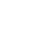Published on

# Top asked JavaScript Interview question and answers | JavaScript interview preparation materialAuthors
•Name
Gyanendra Kumar Knojiya
@gyanknojiya

## 1. What is JSON and its common operations?

JSON is a text-based data format following JavaScript object syntax, which was popularized by Douglas Crockford. It is useful when you want to transmit data across a network and it is just a text file with an extension of .json, and a MIME type of application/JSON

Parsing: Converting a string to a native object

JSON.parse(text)


Stringification: converting a native object to a string so it can be transmitted across the network

JSON.stringify(object)


## 2. What is the purpose of the array slice method

The slice() method returns the selected elements in an array as a new array object. It selects the elements starting at the given start argument and ends at the given optional end argument without including the last element. If you omit the second argument then it selects till the end.

Some of the examples of this method are,

let arrayIntegers = [1, 2, 3, 4, 5]
let arrayIntegers1 = arrayIntegers.slice(0, 2) // returns [1,2]
let arrayIntegers2 = arrayIntegers.slice(2, 3) // returns 
let arrayIntegers3 = arrayIntegers.slice(4) //returns 


## 3. What is the purpose of the array splice method

The splice() method is used to either adds/removes items to/from an array and then return the removed item. The first argument specifies the array position for insertion or deletion whereas the optional second argument indicates the number of elements to be deleted. Each additional argument is added to the array.

Some of the examples of this method are,

let arrayIntegersOriginal1 = [1, 2, 3, 4, 5]
let arrayIntegersOriginal2 = [1, 2, 3, 4, 5]
let arrayIntegersOriginal3 = [1, 2, 3, 4, 5]

let arrayIntegers1 = arrayIntegersOriginal1.splice(0, 2) // returns [1, 2]; original array: [3, 4, 5]
let arrayIntegers2 = arrayIntegersOriginal2.splice(3) // returns [4, 5]; original array: [1, 2, 3]
let arrayIntegers3 = arrayIntegersOriginal3.splice(3, 1, 'a', 'b', 'c') //returns ; original array: [1, 2, 3, "a", "b", "c", 5]


## 4. What is the difference between == and === operators

JavaScript provides both strict(===, !==) and type-converting(==, !=) equality comparison. The strict operators consider the type of variable, while non-strict operators make type correction/conversion based upon values of variables. The strict operators follow the below conditions for different types,

1. Two strings are strictly equal when they have the same sequence of characters, same length, and same characters in corresponding positions.
2. Two numbers are strictly equal when they are numerically equal. i.e, Having the same number value.

i. There are two special cases in this, NaN is not equal to anything, including NaN. ii. Positive and negative zeros are equal 3. Two Boolean operands are strictly equal if both are true or both are false. 4. Two objects are strictly equal if they refer to the same Object. 5. Null and Undefined types are not equal with ===, but equal with ==. i.e, null===undefined --> false but null==undefined --> true

Some of the example which covers the above cases,

0 == false   // true
0 === false  // false
1 == "1"     // true
1 === "1"    // false
null == undefined // true
null === undefined // false
'0' == false // true
'0' === false // false
[]==[] or []===[] //false, refer different objects in memory
{}=={} or {}==={} //false, refer different objects in memory


## 5. What are lambda or arrow functions

An arrow function is a shorter syntax for a function expression and does not have its own this, arguments, super, or new.target. These functions are best suited for non-method functions, and they cannot be used as constructors.

## 6. What is a higher order function

Higher-order function is a function that accepts another function as an argument or returns a function as a return value or both.

const firstOrderFunc = () => console.log('Hello, I am a First order function')
const higherOrder = (returnsFirstOrderFunc) => returnsFirstOrderFunc()
higherOrder(firstOrderFunc)


## 7. What is a unary function

Unary function (i.e. monadic) is a function that accepts exactly one argument. It stands for a single argument accepted by a function.

Let us take an example of unary function,

const unaryFunction = (a) => console.log(a + 10) // Add 10 to the given argument and display the value


## 8. What is a pure function

A Pure function is a function where the return value is only determined by its arguments without any side effects. i.e, If you call a function with the same arguments 'n' number of times and 'n' number of places in the application then it will always return the same value.

Let's take an example to see the difference between pure and impure functions-

//Impure
let numberArray = []
const impureAddNumber = (number) => numberArray.push(number)
//Pure
const pureAddNumber = (number) => (argNumberArray) => argNumberArray.concat([number])
//Display the results
console.log(numberArray) // returns 
console.log(numberArray) // returns 


## 9. What is the difference between let and var

You can list out the differences -

##### var
1. It is been available from the beginning of JavaScript
2. It has function scope
3. Variables will be hoisted
##### let
1. Introduced as part of ES6
2. It has block scope
3. Hoisted but not initialized
function userDetails(username) {
console.log(salary) // undefined due to hoisting
console.log(age) // ReferenceError: Cannot access 'age' before initialization
let age = 30
var salary = 10000
}
console.log(salary) //10000 (accessible to due function scope)
console.log(age) //error: age is not defined(due to block scope)
}
userDetails('John')


## 10. What is Hoisting

Hoisting is a JavaScript mechanism where variables and function declarations are moved to the top of their scope before code execution. Remember that JavaScript only hoists declarations, not initialisation. Let's take a simple example of variable hoisting.

console.log(message) //output : undefined
var message = 'The variable Has been hoisted'


The above code looks like as below to the interpreter-

var message
console.log(message)
message = 'The variable Has been hoisted'